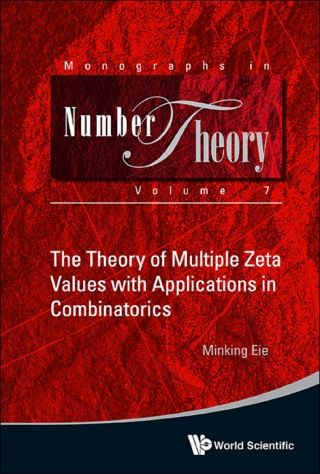## Theory Of Multiple Zeta Values With Applications In Combinatorics, The• Description
• Author
• Info
• Reviews

### Description

This is the first book on the theory of multiple zeta values since its birth around 1994. Readers will find that the shuffle products of multiple zeta values are applied to complicated counting problems in combinatorics, and numerous interesting identities are produced that are ready to be used. This will provide a powerful tool to deal with problems in multiple zeta values, both in evaluations and shuffle relations. The volume will benefit graduate students doing research in number theory.

Contents:
• Basic Theory of Multiple Zeta Values:
• The Time Before Multiple Zeta Values
• Introduction to the Theory of Multiple Zeta Values
• The Sum Formula
• Shuffle Relations among Multiple Zeta Values:
• Some Shuffle Relations
• Euler Decomposition Theorem
• Multiple Zeta Values of Height Two
• Applications of Shuffle Relations in Combinatorics:
• Generalizations of Pascal Identity
• Combinatorial Identities of Convolution Type
• Vector Version of Some Combinatorial Identities
• Appendices:
• Singular Modular Forms on the Exceptional Domain
• Shuffle Product Formulas of Multiple Zeta Values
• The Sum Formula and Their Generalizations